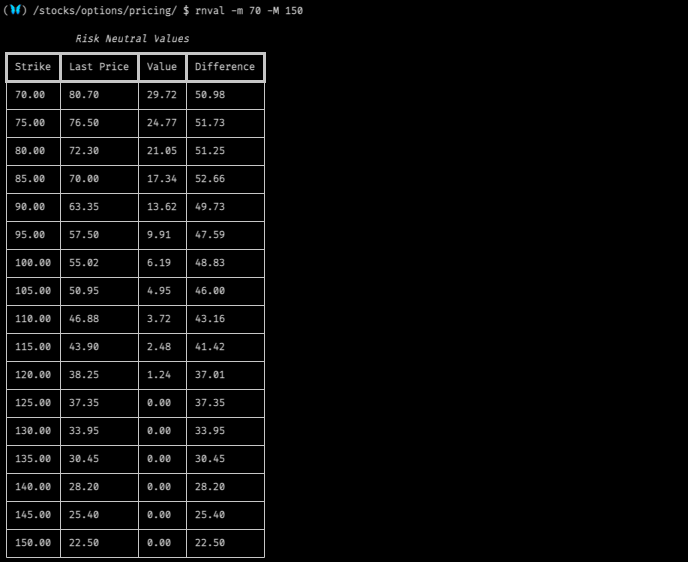# Introduction to the Options Pricing Submenu

This set of features is for composing hypothetical outcomes through user-defined inputs. There are two columns of inputs:

• price - the price for the underlying at the close on expiration.
• probability - the statistical probability of the outcome, where 1 = 100% & probability always = 1.

The Pricing submenu is accessible after selecting an expiration date for the options chain. Type, `pricing`, and press enter for access.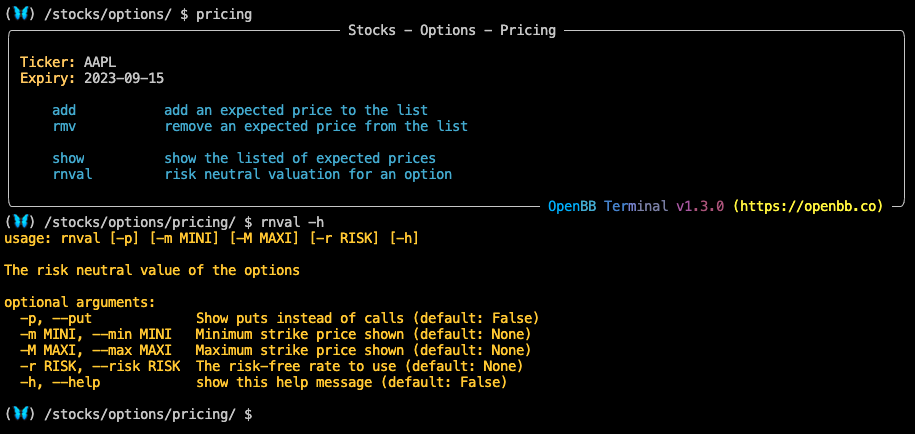## How to use the Pricing Submenu

Use the `add` command to build the list, and use the `rmv` command to take away any entries.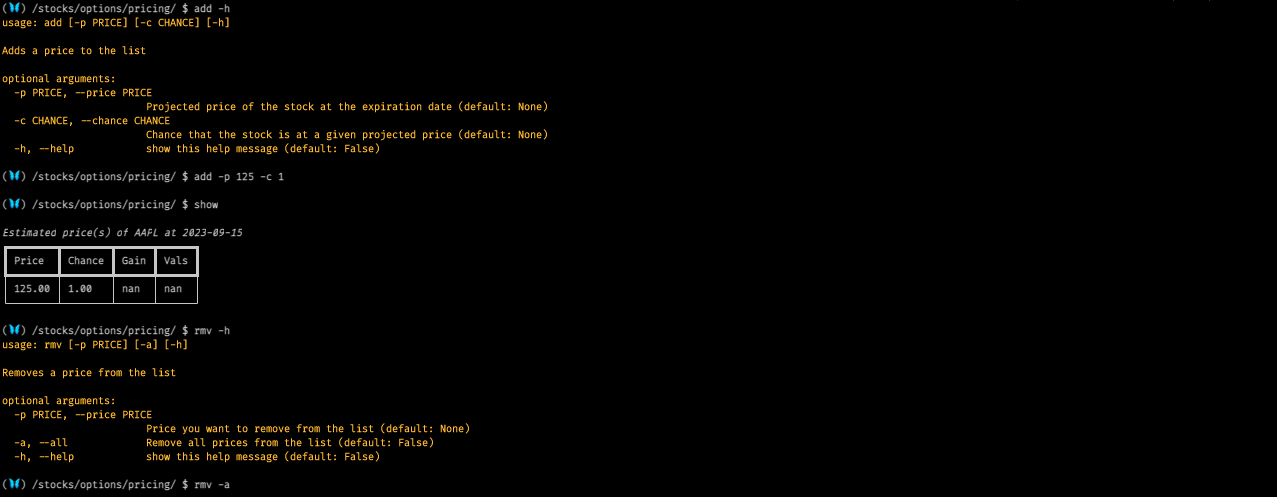`show` will print a table of the inputs. Multiple price points can be added, assuming that probability always sums at 100%.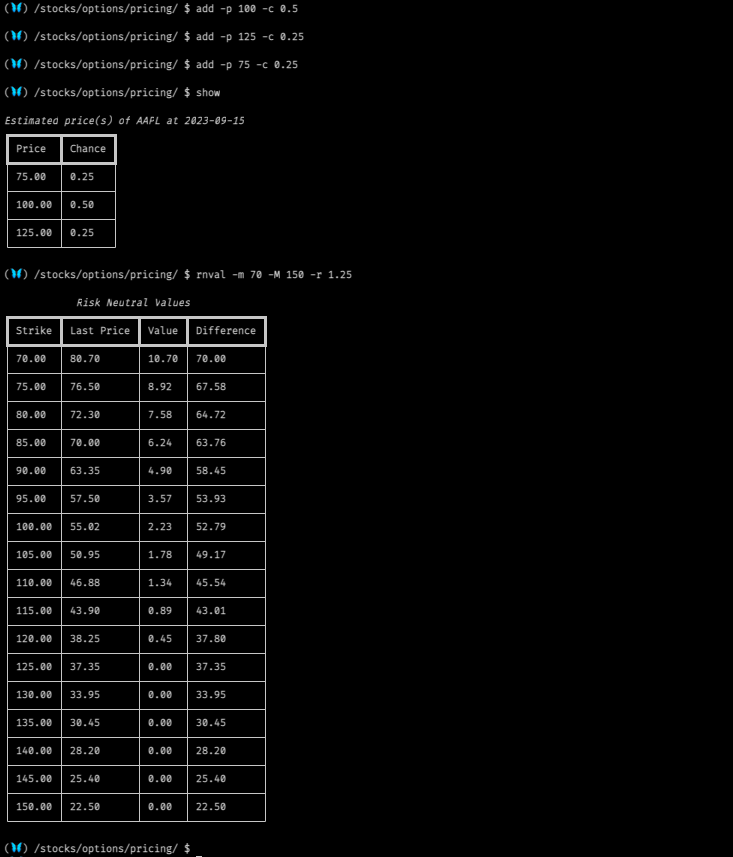## Examples

Adding the optional argument, `-p`, will calculate the puts in the chain.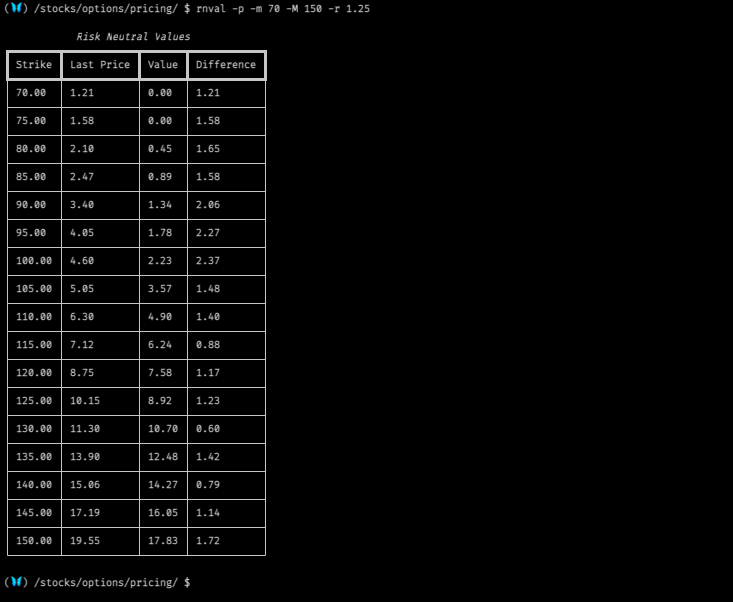Removing the risk-free rate variable makes a substantial difference to the calculated value of an option. With monetary policy on the move, this is an important variable to consider as financial conditions change.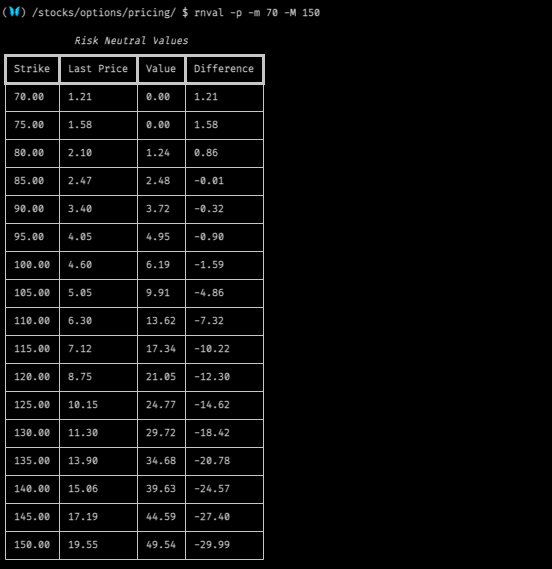RFR as 0 for call options